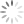Loading, please wait...## Operator Precedence in C

Operator precedence describes that which expression has higher priority than others and which one evaluated first.

Category

Operator

Associativity

Postfix

() [] -> . ++ - -

Left to right

Unary

+ - ! ~ ++ - - (type)* & sizeof

Right to left

Multiplication

* / %

Left to right

+-

Left to right

Shift

<< >>

Left to right

Relational

< <= > >=

Left to right

Equality

== !=

Left to right

Bitwise AND

&

Left to right

Bitwise XOR

^

Left to right

Bitwise OR

|

Left to right

Logical AND

&&

Left to right

Logical OR

||

Left to right

Conditional

?:

Right to left

Assignment

= += -= *= /= %=>>= <<= &= ^= |=

Right to left

Comma

,

Left to right

Example

``````/*A C Program for Operator precedence*/

#include <stdio.h>

main()
{
int num1 = 20;
int num2 = 10;
int num3 = 15;
int num4 = 5;
int num5;
num5= (num1 + num2) * num3 / num4;     // ( 30 * 15 ) / 5
printf("Value of (num1 + num2) * num3 / num4 is : %d\n", num5 );

num5 = ((num1 + num2) * num3) / num4; // (30 * 15 ) / 5
printf("Value of ((num1 + num2) * num3) / num4 is : %d\n" , num5 );

num5 = (num1 + num2) * (num3 / num4); // (30) * (15/5)
printf("Value of (num1 + num2) * (num3 / num4) is : %d\n", num5 );

num5 = num1 + (num2 * num3) / num4; // 20 + (150/5)
printf("Value of num1 + (num2 * num3) / num4 is : %d\n" , num5 );

return 0;
}``````

Output

``````Value of (num1 + num2) * num3 / num4 is : 90

Value of ((num1 + num2) * num3) / num4 is : 90

Value of (num1 + num2) * (num3 / num4) is : 90

Value of num1 + (num2 * num3) / num4 is : 50``````